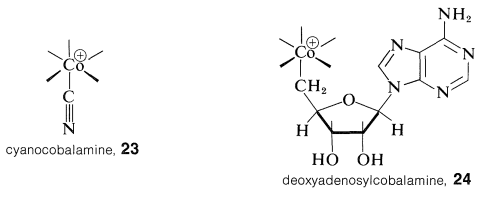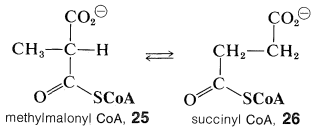# 31.6: Vitamin B₁₂ as an Organometallic Compound

$$\newcommand{\vecs}{\overset { \rightharpoonup} {\mathbf{#1}} }$$ $$\newcommand{\vecd}{\overset{-\!-\!\rightharpoonup}{\vphantom{a}\smash {#1}}}$$$$\newcommand{\id}{\mathrm{id}}$$ $$\newcommand{\Span}{\mathrm{span}}$$ $$\newcommand{\kernel}{\mathrm{null}\,}$$ $$\newcommand{\range}{\mathrm{range}\,}$$ $$\newcommand{\RealPart}{\mathrm{Re}}$$ $$\newcommand{\ImaginaryPart}{\mathrm{Im}}$$ $$\newcommand{\Argument}{\mathrm{Arg}}$$ $$\newcommand{\norm}{\| #1 \|}$$ $$\newcommand{\inner}{\langle #1, #2 \rangle}$$ $$\newcommand{\Span}{\mathrm{span}}$$ $$\newcommand{\id}{\mathrm{id}}$$ $$\newcommand{\Span}{\mathrm{span}}$$ $$\newcommand{\kernel}{\mathrm{null}\,}$$ $$\newcommand{\range}{\mathrm{range}\,}$$ $$\newcommand{\RealPart}{\mathrm{Re}}$$ $$\newcommand{\ImaginaryPart}{\mathrm{Im}}$$ $$\newcommand{\Argument}{\mathrm{Arg}}$$ $$\newcommand{\norm}{\| #1 \|}$$ $$\newcommand{\inner}{\langle #1, #2 \rangle}$$ $$\newcommand{\Span}{\mathrm{span}}$$$$\newcommand{\AA}{\unicode[.8,0]{x212B}}$$

The structure of vitamin B$$_{12}$$ shown in Section 30-6B with a cyanide ion coordinated with cobalt is not the active form of the vitamin but is a particularly stable form, convenient to isolate and handle. The active form is a coenzyme that is remarkable in having a carbon-cobalt bond to an essentially alkyl-type carbon. The carbon-cobalt bond is to a 5'-deoxyadenosyl group, and if we abbreviate vitamin B$$_{12}$$ coordinated to cyanide as $$23$$, the coenzyme can be written, in the same style, as $$24$$. (You will notice that $$23$$ is an abbreviation of the formula of Section 30-6B turned $$180^\text{o}$$.)Both $$23$$ and the B$$_{12}$$ coenzyme, $$24$$, are compounds of $$\ce{Co}$$(III) and both substances have all electrons paired. B$$_{12}$$ can be reduced to a form with $$\ce{Co}$$(II) which has an unpaired electron and gives an ESR signal (Section 27-9). The cobalt-carbon bond of $$24$$ appears to be formed from $$23$$ by removal of the cyano group and a two-electron reduction to $$\ce{Co}$$(I). The reduced cobalt is powerfully nucleophilic and probably is alkylated with adenosine triphosphate (ATP, Section 15-5F) to form $$24$$:Vitamin B$$_{12}$$ coenzyme participates in several biological reactions but none is more unusual, or as hard to rationalize, as its role in the interconversion of methylpropanedioyl CoA (methylmalonyl CoA, $$25$$) to butanedioyl CoA (succinyl CoA, $$26$$):$$^3$$This rearrangement, which is important in the biochemical utilization of propanoic acid, has been shown to involve transfer of a hydrogen from the $$\ce{CH_3}-$$ group of $$25$$ to the $$\ce{-CH_2R}$$ group of $$24$$. Then rearrangement and formation of $$26$$ occurs along with reformation of $$24$$:We formulate the intermediate oxidized forms of $$25$$ and $$26$$ with cobalt-to-carbon bonds, but there is no definitive evidence that this is correct. The overall reaction involves attack on the $$\ce{CH_3}-$$ of $$25$$, not an easy reaction to carry out in the laboratory, except with reagents such as $$\ce{Cl} \cdot$$, because this $$\ce{CH_3}$$ is not adjacent to a double bond or other activating group. Furthermore, there is no very good analogy for the rearrangement step. At present, although it is known that $$24$$ is reduced to give $$\ce{CH_3-R}$$, the details of this important biochemical mechanism remain to be elucidated by further research.

$$^3$$For the structure of CoA, see Section 18-8F.